#### Algorithm execution

Figures 6.6 and 6.7 show how the algorithm proceeds. Initially,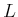is empty, and a doubly connected edge list is used to represent. Each connected component ofyields a single face in the data structure. Suppose inductively that after several events occur,is correctly maintained. For each event, one of the four cases in Figure 6.2 occurs. By maintainingin a balanced binary search tree , the edges above and below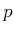can be determined in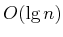time. This is much better than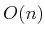time, which would arise from checking every segment. Depending on which of the four cases from Figure 6.2 occurs, different updates toare made. If the first case occurs, then two different edges are inserted, and the face of whichis on the border is split two times by vertical line segments. For each of the two vertical line segments, two half-edges are added, and all faces and half-edges must be updated correctly (this operation is local in that only records adjacent to where the change occurs need to be updated). The next two cases in Figure 6.2 are simpler; only a single face split is made. For the final case, no splitting occurs.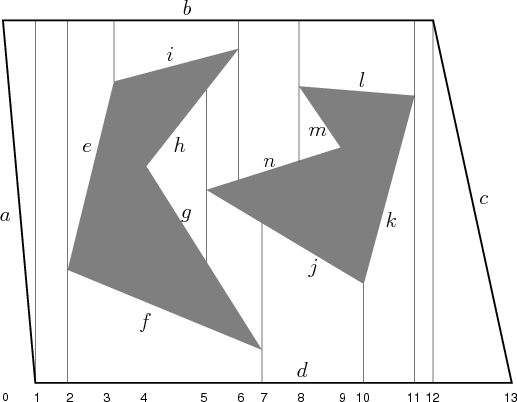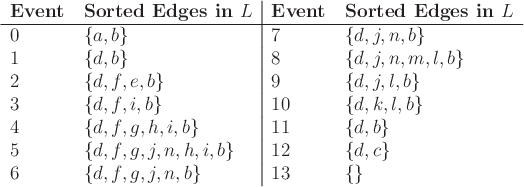Once the face splitting operations have been performed,needs to be updated. When the sweep line crosses, two edges are always affected. For example, in the first and last cases of Figure 6.2, two edges must be inserted into(the mirror images of these cases cause two edges to be deleted from). If the middle two cases occur, then one edge is replaced by another in. These insertion and deletion operations can be performed intime. Since there areevents, the running time for the construction algorithm is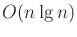.

The roadmap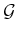can be computed from the face pointers of the doubly connected edge list. A more elegant approach is to incrementally buildat each event. In fact, all of the pointer maintenance required to obtain a consistent doubly connected edge list can be ignored if desired, as long asis correctly built and the sample point is obtained for each cell along the way. We can even go one step further, by forgetting about the cell decomposition and directly building a topological graph of line-segment paths between all sample points of adjacent cells.

Steven M LaValle 2020-08-14